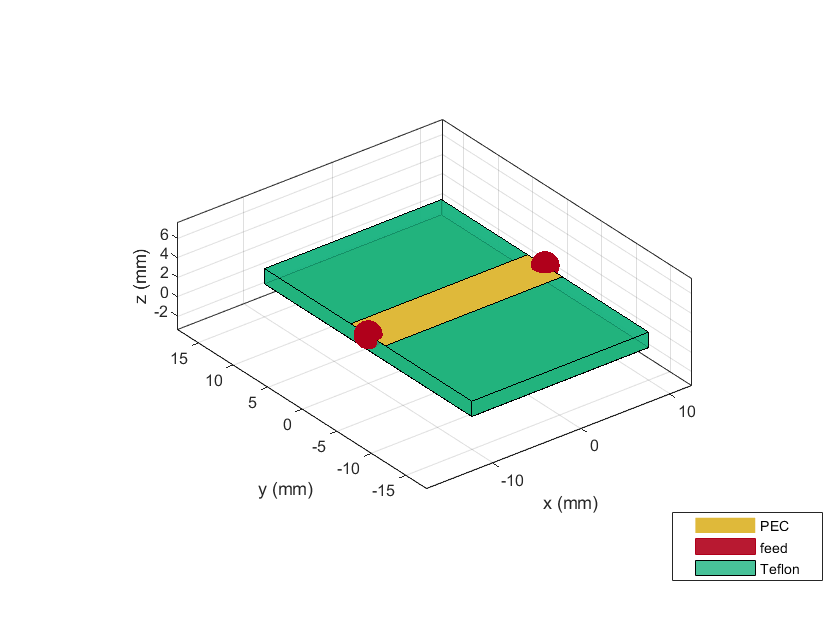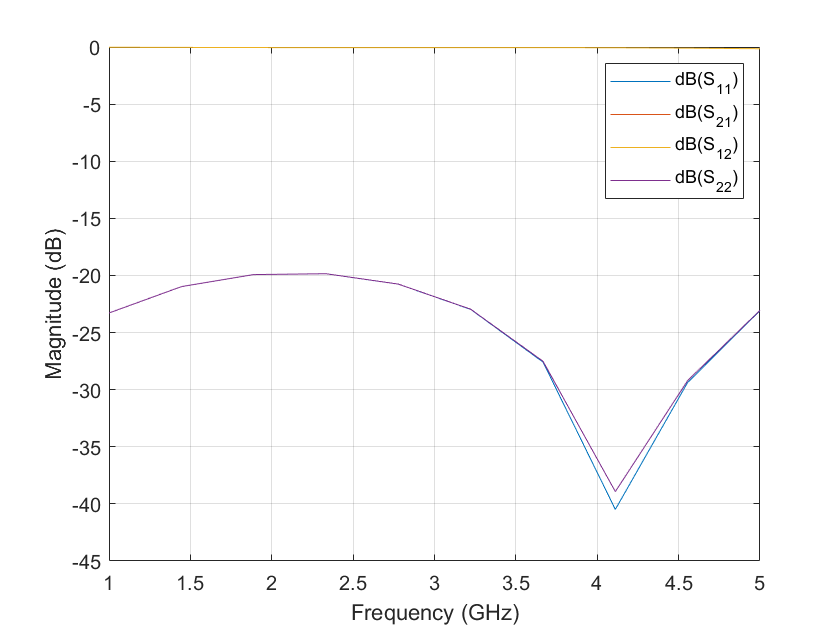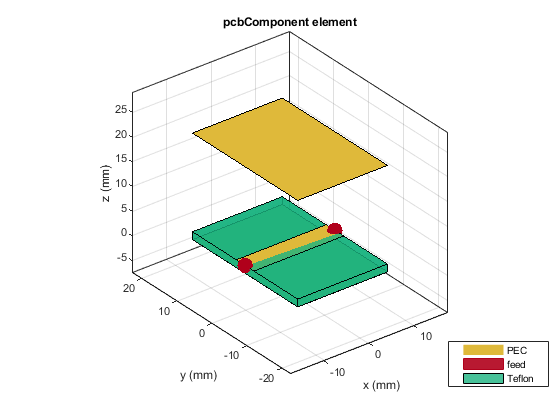pcbComponent

Create single or multifeed PCB component

Description

Use the pcbComponent object to create a multiport PCB component consisting of metal and dielectric layers.

Creation

Description

example

pcb = pcbComponent creates a two-port PCB component.

pcb = pcbComponent(Name=Value) sets Properties using one or more name-value arguments. For example, pcb = pcbComponent(Name=PCBWilkinson) creates a PCB component named 'PCBWilkinson' Properties not specified retain their default values.

Properties

expand all

Name of the PCB component, specified a character vector or string scalar.

Example: component = pcbComponent(Name='PCBsplitter')

Data Types: char | string

Design revision details of the PCB component, specified as a character vector or string scalar.

Example: component = pcbComponent(Revision='v2.0')

Data Types: char | string

Shape of the PC board, specified as a shape object. You can specify any one of the shapes from Custom Geometry and PCB Fabrication.

Example: trace = tracerectangular; component = pcbComponent(BoardShape=trace) creates a rectangle shaped trace on a PCB.

Data Types: char | string

Height of the PCB component, specified as a positive scalar in meters.

Example: component = pcbComponent(BoardThickness=0.0026)

Data Types: double

Metal and dielectric layers, specified a cell array of metal and dielectric layers. You can specify one metal shape or one dielectric substrate per layer starting with the top layer and proceeding downward.

Data Types: cell

Feed locations on the PCB component in Cartesian coordinates, specified as either an N-by-3 or N-by-4 array with N representing the number for ports on the PCB component. You can place the feed inside the board or at the edge of the board. The arrays translate into the following:

• N-by-3 – [x, y, Layer]

• N-by-4 – [x, y, SigLayer, GndLayer]

Example: component = pcbComponent(FeedLocations=[-0.0187 0 1 2])

Data Types: double

Diameter of center pin of the feed connector, specified as a positive scalar in meters.

Example: component = pcbComponent(FeedDiameter=2.000e-04)

Data Types: double

Electrical short locations on the PCB component in Cartesian coordinates, specified as a real vector of size M-by-4 array. The array translates into the following:

• M-by-4 – [x, y, SigLayer, GndLayer]

Example: component = pcbComponent(ViaLocations=[0 -0.025 1 2])

Data Types: double

Diameter of electrical shorting pin used between metal layers, specified as a positive scalar in meters.

Data Types: double

Model for approximating the feed and via, specified as one of the following:

• 'strip' – A rectangular strip approximation to the feed and via cylinder. This approximation is the simplest and results in a small mesh.

• 'square' – A four-sided polyhedron approximation to the feed and via cylinder.

• 'hexagon' – A six-sided polyhedron approximation to the feed and via cylinder.

• 'octagon' – A eight-sided polyhedron approximation to the feed and via cylinder.

Example: component = pcbComponent(FeedViaModel='octagon')

Data Types: char | string

Type of the metal used as a conductor, specified as a metal object. You can choose any metal from the MetalCatalog or specify a metal of your choice. For more information, see metal. For more information on metal conductor meshing, see Method of Moments Solver for Metal and Dielectric Structures.

Example: m = metal('Copper'); component = pcbComponent(Conductor=m)

Lumped elements added to the PCB component feed, specified as a lumped element object handle. For more information, see lumpedElement (Antenna Toolbox) Antenna Toolbox™.

Example: Load = lumpedelement. lumpedelement is the object handle for the load created using lumpedElement.

Tilt angle of the PCB component, specified as a scalar or vector with each element unit in degrees.

Example: Tilt=90

Example: pcb.Tilt = 90

Example: Tilt=[90 90],TiltAxis=[0 1 0;0 1 1] tilts the PCB component at 90 degrees about the two axes defined by the vectors.

Data Types: double

Tilt axis of the PCB component, specified as:

• Three-element vector of Cartesian coordinates in meters. In this case, each coordinate in the vector starts at the origin and lies along the specified points on the X-, Y-, and Z-axes.

• Two points in space, each specified as three-element vectors of Cartesian coordinates. In this case, the PCB component rotates around the line joining the two points in space.

• A string input describing simple rotations around one of the principal axes, 'X', 'Y', or 'Z'.

Example: TiltAxis=[0 1 0]

Example: TiltAxis=[0 0 0;0 1 0]

Example: pcb.TiltAxis = 'Z'

Data Types: double

Object Functions

 current Calculate and plot current distribution charge Calculate and plot charge distribution feedCurrent Calculate current at feed port gerberWrite Generate Gerber files layout Plot all metal layers and board shape mesh Change and view mesh properties of metal or dielectric in PCB component meshconfig Change mesh mode of PCB component or shape structure sparameters Calculate S-parameters for RF PCB objects show Display PCB component structure or PCB shape

Examples

collapse all

Create a PCB component using default properties.

pcb = pcbComponent;

View the PCB component.

show(pcb)Calculate S-parameters over 10 frequencies from 1-5 GHz.

s=sparameters(pcb,linspace(1e9,5e9,10));

Plot the S-parameters.

rfplot(s)Create a PCB component with a lid at a distance of 2 cm above the component.

p = pcbComponent;
pcblid = antenna.Rectangle(Length=p.Layers{1}.Length,Width=p.Layers{3}.Width);
dAir = dielectric('Air');
dAir.Thickness = 2e-2;
p.BoardThickness = p.BoardThickness + dAir.Thickness;
p.Layers = {pcblid,dAir,p.Layers{1},p.Layers{2},p.Layers{3}};
p.FeedLocations(:,3:4) = [3 5;3 5];
show(p)Calculate the S-parameters over the 10 frequencies from 1-5 GHz.

s = sparameters(p,linspace(1e9,5e9,10));